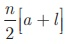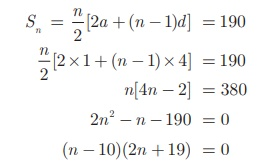Home | | Maths 10th Std | Series: Sum to n terms of an A.P.(Arithmetic Progression)

# Series: Sum to n terms of an A.P.(Arithmetic Progression)

If a series has finite number of terms then it is called a Finite series. If a series has infinite number of terms then it is called an Infinite series. Let us focus our attention only on studying finite series.

## Series

The sum of the terms of a sequence is called series. Leta1, a2, a3,..., an ,... be the sequence of real numbers. Then the real number a1 + a2 + a3 + is defined as the series of real numbers.

If a series has finite number of terms then it is called a Finite series. If a series has infinite number of terms then it is called an Infinite series. Let us focus our attention only on studying finite series

## Sum to n terms of an A.P.

A series whose terms are in Arithmetic progression is called Arithmetic series.

Let a , a + d , a + 2d , a + 3d,... be the Arithmetic Progression.

The sum of first n terms of a Arithmetic Progression denoted by Sn is given by,

Sn=a +(a + d ) +(a + 2d ) +  + (a +(n −1)d )                 …….(1)

Rewriting the above in reverse order

Sn =(a +(n − 1)d) +(a + (n −2)d ) +  +(a + d ) +a         ..(2)

Adding (1) and (2) we get,

2S n  =[a +a + (n 1)d ]+[ a +d +a +(n 2)d ] ++ [a +(n2)d +(a +d )]+[a + (n1)d +a]

= [2a +(n1)d ] +[2a + (n1)d + .. .. +[2a + (n1)d ]    (n terms)

2Sn= n ×[2a + (n1)d ]      gives Sn  = n/2 [2a + (n 1)d ]

Note

If the first term a, and the last term l (nth  term) are given thenExample 2.31 Find the sum of first 15 terms of the A. P.Solution

Here the first term a = 8, common difference d  =Sum of first n terms of an A.P.### Example 2.32

Find the sum of 0. 40 + 0. 43 + 0. 46 + + 1 .

### Solution

Here the value of n is not given. But the last term is given. From this, we can find the value of n.

Given a = 0. 40 and l = 1 , we find d= 0. 43 − 0.40 = 0. 03 .

Therefore,Sum of first n terms of an A.P. SnHere, n = 21 .  Therefore,So, the sum of 21 terms of the given series is 14.7.

### Example 2.33

How many terms of the series 1 + 5 + 9 + ... must be taken so that their sum is 190?

### Solution

Here we have to find the value of n, such that Sn  = 190.

First term a = 1, common difference d = 5 −1 = 4 .

Sum of first n terms of an A.P.But n = 10 as n = −19/2 is impossible. Therefore, n = 10 .

State True or False. Justify it.

1. The nth term of any A.P. is of the form pn+q where p and q are some constants.

2. The sum to nth term of any A.P. is of the form pn2+qn + r where p, q, r are some constants.

### Example 2.34

The 13th term of an A.P. is 3 and the sum of first 13 terms is 234. Find the common difference and the sum of first 21 terms.

### Solution

Given the 13th term = 3 so, t13  = a + 12d = 3             .........(1)

Sum of first 13 terms = 234  gives S 132a + 12d = 36             .........(2)

Solving (1) and (2) we get, a = 33, d = −5 /2

Therefore, common difference is -5/2

Sum of first 21 terms = S21### Example 2.35

In an A.P. the sum of first n terms is 5n2/2 + 3n/2 . Find the 17th term.

Solution The 17th term can be obtained by subtracting the sum of first 16 terms from the sum of first 17 terms.### Example 2.36

Find the sum of all natural numbers between 300 and 600 which are divisible by 7.

### Solution

The natural numbers between 300 and 600 which are divisible by 7 are 301, 308, 315, …, 595.

The sum of all natural numbers between 300 and 600 is 301 + 308 + 315 + + 595.

The terms of the above series are in A.P.

First term a = 301 ; common difference d = 7 ; Last term l = 595.Since, Sn  = n/2 [a +l ] , we have S57  = 43/2 [ 301 + 595]= 19264.

### Example 2.37

A mosaic is designed in the shape of an equilateral triangle, 12 ft on each side. Each tile in the mosaic is in the shape of an equilateral triangle of 12 inch side. The tiles are alternate in colour as shown in the figure. Find the number of tiles of each colour and total number of tiles in the mosaic.### Solution

Since the mosaic is in the shape of an equilateral triangle of 12 ft, and the tile is in the shape of an equilateral triangle of 12 inch (1 ft), there will be 12 rows in the mosaic

From the figure, it is clear that number of white tiles in each row are 1, 2, 3, 4, …, 12 which clearly forms an Arithmetic Progression.

Similarly the number of blue tiles in each row are 0, 1, 2, 3, …, 11 which is also an Arithmetic Progression.

Number of white tiles = 1 + 2 + 3 + … + 12 = 12/2 [1 + 12] = 78

Number of blue tiles = 0 + 1 + 2 + 3 + … + 11 = 12/2  [0 + 11] = 66

The total number of tiles in the mosaic = 78 + 66 = 144

### Example 2.38

The houses of a street are numbered from 1 to 49. Senthil’s house is numbered such that the sum of numbers of the houses prior to Senthil’s house is equal to the sum of numbers of the houses following Senthil’s house. Find Senthil’s house number?

### Solution

Let Senthil’s house number be x.

It is given thatx2 =2450 −x 2x 2x 2  = 2450

x2 =1225 gives x = 35

Therefore, Senthil’s house number is 35.

Example 2.39

The sum of first n, 2n and 3n terms of an A.P. are S1 ,S2 and S3 respectively.

Prove that S3  = 3(S2S1 ).

### Solution

If S1 ,S2 and S3 are sum of first n, 2n and 3n terms of an A.P. respectively thenTags : Example, Solution | Mathematics , 10th Mathematics : UNIT 2 : Numbers and Sequences
Study Material, Lecturing Notes, Assignment, Reference, Wiki description explanation, brief detail
10th Mathematics : UNIT 2 : Numbers and Sequences : Series: Sum to n terms of an A.P.(Arithmetic Progression) | Example, Solution | Mathematics